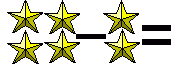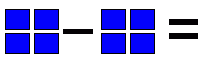Email us to get an instant 20% discount on highly effective K-12 Math & English kwizNET Programs!

#### Online Quiz (WorksheetABCD)

Questions Per Quiz = 2 4 6 8 10

### Grade 1 - Mathematics3.6 Practice Subtraction Using Pictures

 Method: Count the number on each side. Subtract the numbers Example: Subtract from the figure and select the correct answer.Count the number of patterns on each side From the above pattern we have 4 - 3 Answer: 1 Directions: Answer the following questions. Also write at least ten examples of your own.
 Q 1: Subtract from the figure and write the correct answer.Answer: Q 2: Subtract from the figure and write the correct answer.Answer: Q 3: Subtract from the figure and write the correct answer.Answer: Q 4: Subtract from the figure and write the correct answer.Answer: Q 5: Subtract from the figure and write the correct answer.Answer: Q 6: Subtract from the figure and write the correct answer.Answer: Question 7: This question is available to subscribers only! Question 8: This question is available to subscribers only!